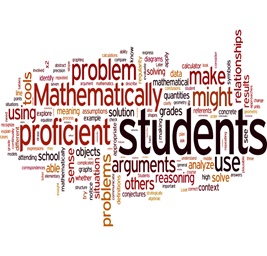## MATH Resource SiteThe resources posted on this website are for parents and students to use as needed. These resources were created to give parents and students a better understanding of the math concepts presented in classrooms.

Common Core Math Videos

An Introduction to Sprints

Sprints

Pictorial Representation of the Array Pattern

Arrays

Arrays with Number Disks

Arrays with Fractions

Array Model Progressions

Concrete Representation of the Array Pattern

Concrete Representation of the Array Pattern V2

Intro to Place Value with Number Disks

General Story of Units Number Disks

Subtraction Problems Using Number Disks

Decimal Subtraction Problems Using Number Disks

Multiplication Algorithms Using Number Disks

Division Algorithms Using Number Disks

Vertical Number Line Rounding

Progression of Fractions Through Number Lines

Progression of Multiplication and Division Tape Diagrams

Tape Diagrams/ Word Problems

Tape Diagrams Grade 5

Number Bonds (Full Length)

Progression of Number Bonds: Grades 3-5

Number Bond Decomposition: Grades 3-5

Number Bond Decomposition: Fractions

Number Bond Decomposition: Multiplication

Number Bond Decomposition with Mixed Units

Number Bond Decomposition: Division and Multiplication Algorithms (Grades 3-5)

Choral Response